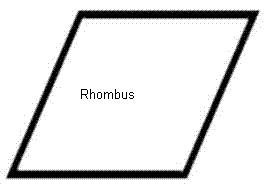# Rhombus Calculator

Calculate the various properties of rhombus for given values.

Area of Rhombus [Base Times Height Method] : [ base×height ]

Enter the lengths of base and height

 Enter Base : Enter height :
 Area of Rhombus =

Area of Rhombus [Diagonal Method] : [ ½×d1d2 ]

Enter the Diagonal lengths d1 and d2

 Enter the Diagonal d1 : Enter the Diagonal d2 :
 Area of Rhombus =

Area of Rhombus Using Trigonometry : [ (side)² Sin A ]
 Enter the length : Enter the angle : Area of Rhombus =

Perimeter of Rhombus : [ 4(side) ]
 Enter the length : Perimeter of Rhombus =

## Rhombus

The rhombus is a special type of parallelogram having the opposite angles are equal and opposite sides are parallel. Rhombus has four sided flat shape and all four sides are having same length. The rhombuses fall under the category of quadrilaterals (a flat shape with four sides).### Formula used

#### Area of Rhombus

[Base Times Height Method] : [ base×height ]

[Diagonal Method] : [ ½×d1d2 ]

[Trigonometry Method]: [ (side)² Sin A ]

#### Perimeter of Rhombus

Perimeter of Rhombus : [ 4(side) ]